# Chapter 9 Circles NCERT Exemplar Solutions Exercise 9.4 Class 10 Maths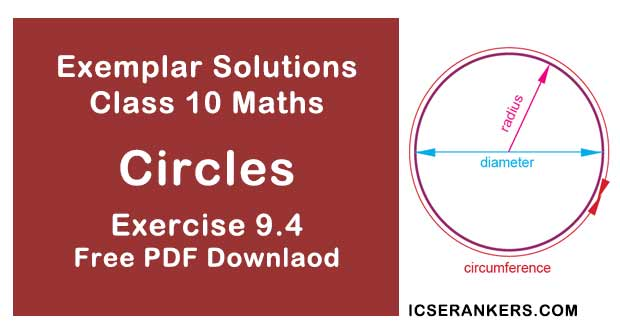Chapter Name NCERT Maths Exemplar Solutions for Chapter 9 Circles Exercise 9.4 Book Name NCERT Exemplar for Class 10 Maths Other Exercises Exercise 9.1Exercise 9.2Exercise 9.3 Related Study NCERT Solutions for Class 10 Maths

### Exercise 9.4 Solutions

1. If a hexagon ABCDEF circumscribe a circle, prove that AB + CD + EF = BC + DE + FA.

Solution

To prove:
AB + CD + EF = BC + DE + FA
We know that,
Tangents drawn from an external point to a circle are equal.
So,
AM = RA  ...i [tangents from point A]
BM = BN  ...ii [tangents from point B]
CO = NC  ...iii [tangents from point C]
OD = DP  ...iv [tangents from point D]
EQ = PE  ...v [tangents from point E]
QF = FR  ...vi [tangents from point F]
[eq i]+[eq ii]+[eq iii]+[eq iv]+[eq v]+[eq vi]
AM + BM + CO + OD + EQ + QF = RA + BN + NC + DP + PE + FR
On solving, we get,
(AM + BM) + (CO + OD) + (EQ + QF) = (BN + NC) + (DP + PE) + (FR + RA)
⇒ AB + CD + EF = BC + DE + FA
Hence Proved!

2. Let s denote the semi-perimeter of a triangle ABC in which BC = a, CA = b, AB = c. If a circle touches the sides BC, CA, AB at D, E, F, respectively, prove that BD = s – b.

Solution

As given in the question,
A triangle ABC with BC = a , CA = b and AB = c . Also, a circle is inscribed which touches the sides BC, CA and AB at D, E and F respectively and s is semi- perimeter of the triangle
To Prove:
BD = s – b
We have,
Semi Perimeter = s
Perimeter = 2s
2s = AB + BC + AC  ...(i)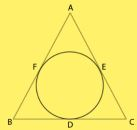We know that,
Tangents drawn from an external point to a circle are equal
So,
AF = AE  ...[ii] [Tangents from point A]
BF = BD  ...[iii] [Tangents From point B]
CD = CE  ...[iv] [Tangents From point C]
AF + BF + CD = AE + BD + CE
⇒ AB + CD = AC + BD
AB + CD + BD = AC + BD + BD
⇒ AB + BC – AC = 2BD
⇒ AB + BC + AC – AC – AC = 2BD
⇒ 2s – 2AC = 2BD  [From i]
⇒ 2BD = 2s – 2b  [as AC = b]
⇒ BD = s – b
Hence Proved.

3. From an external point P, two tangents, PA and PB are drawn to a circle with centre O. At one point E on the circle tangent is drawn which intersects PA and PB at C and D, respectively. If PA = 10 cm, find the perimeter of the triangle PCD.

Solution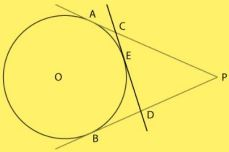As given in the question,
From an external point P, two tangents, PA and PB are drawn to a circle with center O. At a point E on the circle tangent is drawn which intersects PA and PB at C and D, respectively.
And PA = 10 cm
To Find : Perimeter of △PCD
As we know that, Tangents drawn from an external point to a circle are equal.
So, we have,
AC = CE ...[i] [Tangents from point C]
ED = DB ...[ii] [Tangents from point D]
Now,
Perimeter of Triangle PCD = PC + CD + DP
= PC + CE + ED + DP
= PC + AC + DB + DP ...[From i and ii]
= PA + PB
Now,
PA = PB = 10 cm as tangents drawn from an external point to a circle are equal
So ,
Perimeter = PA + PB
= 10 + 10
= 20 cm

4. If AB is a chord of a circle with centre O, AOC is a diameter and AT is the tangent at A as shown in Fig.. Prove that ∠BAT = ∠ACB.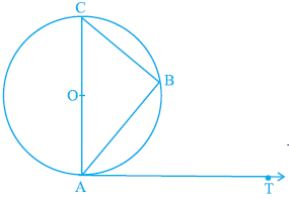Solution

As given in the question,
A circle with center O and AC as a diameter and AB and BC as two chords also AT is a tangent at point A
To Prove : ∠BAT = ∠ACB
Proof :
∠ABC = 90° [Angle in a semicircle is a right angle]
In △ABC By angle sum property of triangle
∠ABC + ∠ BAC + ∠ACB = 180 °
⇒ ∠ACB + 90° = 180° – ∠BAC
⇒ ∠ACB = 90 – ∠BAC ...[i]
Now,
OA ⏊ AT
[Tangent at a point on the circle is perpendicular to the radius through point of contact]
∠OAT = ∠CAT = 90°
⇒ ∠BAC + ∠BAT = 90°
⇒ ∠BAT = 90° – ∠BAC [ii]
From [i] and [ii],
∠BAT = ∠ACB  [Proved]

5. Two circles with centres O and O‘ of radii 3 cm and 4 cm, respectively intersect at two points P and Q such that OP and O‘P are tangents to the two circles. Find the length of the common chord PQ.

Solution

We have,
Two circles with centers O and O’ of radii 3 cm and 4 cm, respectively intersect at two points P and Q, such that OP and O’P are tangents to the two circles and PQ is a common chord.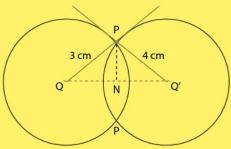To Find: Length of common chord PQ
∠OPO’ = 90°
[Tangent at a point on the circle is perpendicular to the radius through point of contact]
Therefore,
OPO is a right-angled triangle at P
By Pythagoras in △ OPO’, we have
(OO’)2 = (O’P)2 + (OP)2
⇒ (OO’)2 = (4)2 + (3)2
⇒ (OO’)2 = 25
⇒ OO’ = 5 cm
Let ON = x cm and
NO’ = 5 – x cm
In right angled triangle ONP
(ON)2 + (PN)2 = (OP)2
⇒ x2 + (PN)2 = (3)2
⇒ (PN)2  = 9 – x2 ...[i]
In right angled triangle O’NP
(O’N)2 + (PN)2 = (O’P)2
⇒ (5 – x)2 + (PN)2 = (4)2
⇒ 25 – 10x + x2 + (PN)2 = 16
⇒ (PN)2 = -x2  + 10x – 9 ...[ii]
From [i] and [ii]
9 – x2 = -x2 + 10x – 9
⇒ 10x = 18 x = 1.8
From (1) we have
(PN)2 = 9 – (1.8)2
= 9 – 3.24
= 5.76
PN = 2.4 cm
PQ = 2PN
= 2(2.4)
= 4.8 cm

6. In a right triangle ABC in which ∠B = 90°, a circle is drawn with AB as diameter intersecting the hypotenuse AC and P. Prove that the tangent to the circle at P bisects BC.
Solution
As given in the question,
In a right angle ΔABC is which ∠B = 90°, a circle is drawn with AB as diameter intersecting
the hypotenuse AC at P. Also PQ is a tangent at P
To Prove:
PQ bisects BC or, BQ = QC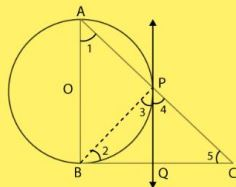We have,
∠APB = 90°  [Angle in a semicircle is a right-angle]
∠BPC = 90°  [Linear Pair]
∠3 + ∠4 = 90  ...[i]
Now,
∠ABC = 90°
In △ABC,
∠ABC + ∠BAC + ∠ACB = 180°
⇒ 90 + ∠1 + ∠5 = 180
⇒ ∠1 + ∠5 = 90  ...[ii]
Now,
∠ 1 = ∠ 3
[angle between tangent and the chord equals angle made by the chord in alternate segment]
Using this in [ii] we have,
∠3 + ∠5 = 90  ...[iii]
From [i] and [iii] we have
∠3 + ∠4 = ∠3 + ∠5
⇒ ∠4 = ∠5
⇒ QC = PQ  [Sides opposite to equal angles are equal]
Also
PQ = BQ  [Tangents drawn from an external point to a circle are equal]
So,
BQ = QC
Therefore, PQ bisects BC

7. In Fig., tangents PQ and PR are drawn to a circle such that ∠RPQ = 30°. A chord RS is drawn parallel to the tangent PQ. Find the ∠RQS.
[Hint: Draw a line through Q and perpendicular to QP.]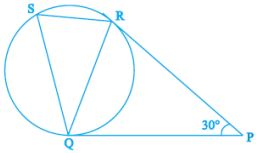Solution
As given in the question,
Tangents PQ and PR are drawn to a circle such that ∠RPQ = 30°. A chord RS is drawn
parallel to the tangent PQ.
To Find :
∠RQS
PQ = PR  [Tangents drawn from an external point to a circle are equal]
∠PRQ = ∠PQR  [Angles opposite to equal sides are equal] ..[i]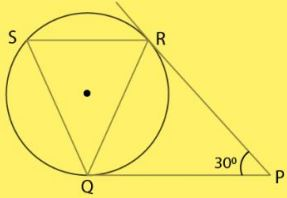In △PQR
∠PRQ + ∠PQR + ∠QPR = 180°
⇒ ∠PQR + ∠PQR + ∠QPR = 180° [Using 1]
⇒ 2∠PQR + ∠RPQ = 180°
⇒ 2∠PQR + 30 = 180
⇒ 2∠PQR = 150
⇒ ∠PQR = 75°
∠QRS = ∠PQR = 75°  [Alternate interior angles]
⇒ ∠QSR = ∠PQR = 75°
[angle between tangent and the chord equals angle made by the chord in alternate segment]
Now
In △RQS
∠RQS + ∠QRS + ∠QSR = 180
⇒ ∠RQS + 75 + 75 = 180
⇒ ∠RQS = 30°

8. AB is a diameter and AC is a chord of a circle with centre O such that ∠BAC 30° . The tangent at C intersects extended AB at a point D.
Prove that BC = BD.
Solution
Given,
AB is a diameter and AC is a chord of circle with centre O, ∠BAC = 30°
To prove : BC = BD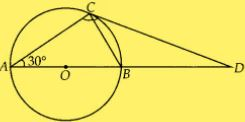Construction: Join BC
∠BCD = ∠CAB  [Angles in alternate segment]
∠CAB = 30°  [Given]
∠BCD = 30° ...(i)
∠ACB = 90° [Angle in semi-circle]
In ∆ABC,
∠A + ∠B + ∠C = 180°  [Angle sum property]
⇒ 30° + ∠CBA + 90° = 180°
⇒ ∠CBA = 60°
Also,
∠CBA + ∠CBD = 180°  [Linear pair]
⇒ ∠CBD = 180° – 60° = 120°
[as, ∠CBA = 60°]
Now,
In ACBD,
∠CBD + ∠BDC + ∠DCB = 180°
⇒ 120° + ∠BDC + 30° = 180°
⇒ ∠BDC = 30° ...(ii)
From (i) and (ii),
∠BCD = ∠BDC
BC = BD
[Sides opposite to equal angles are equal]

9. Prove that the tangent drawn at the mid-point of an arc of a circle is parallel to the chord joining the end points of the arc.
Solution
Let us take the mid-point of an arc AMB be M and TMT’ be the tangent to the circle.
Join AB, AM and MB.
Since,
arc AM = arc MB =3
Chord AM = Chord MB
In ∆AMB,
AM = MB
∠MAB = ∠MBA  ...(i)
[Sides opposite to equal angles are equal]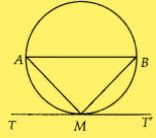Since, TMT’ is a tangent line.
Therefore,
∠AMT = ∠MBA  [Angles in alternate segments are equal]
= ∠MAB [from equation (i)]
But, ∠AMT and ∠MAB are alternate angles, which is possible only when AB is parallel to
TMT
Hence, the tangent drawn at the mid-point of an arc of a circle is parallel to the chord joining
the end points of the arc.

10. In Fig., the common tangent, AB and CD to two circles with centres O and O' intersect at E. Prove that the points O, E, O' are collinear.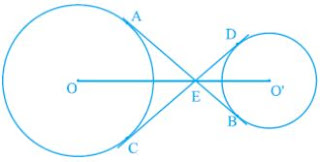Solution
Join AO, OC and O’D, O’B.
Now,
In ∆EO’D and ∆EO’B,
O’D = O’B
O’E = O’E
ED = EB
[Tangents drawn from an external point to the circle are equal in length]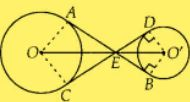EO’D ≅ ∆ EO’B  [By SSS congruence criterion]
∠O’ED = ∠O’EB ...(i)
Therefore,
O’E is the angle bisector of ∠DEB.
Similarly,
OE is the angle bisector of ∠AEC.
∠O’DE = ∠O’BE = 90°
[CED is a tangent to the circle and O’D is the radius, i.e., O’D ⊥ CED]
∠O’DE + ∠O’BE = 180°
∠DEB + ∠DO’B = 180°
[as, DEBO’ is cyclic quadrilateral] ...(ii)
Since,
AB is a straight line.
∠AED + ∠DEB = 180°
⇒ ∠AED + 180° – ∠DO’B = 180° [from (ii)]
⇒ ∠AED = ∠DO’B ...(iii)
Similarly,
∠AED = ∠AOC ...(iv)
Again from eq. (ii),
∠DEB = 180° – ∠DO’B
Dividing by 2 on both sides, we get
(1/2) ∠DEB = 90° - (1/2)∠DO'B
⇒ ∠DEO' = 90° - (1/2)∠DO'B ...(v)
Similarly,
∠AEC = 180° - AOC
Dividing 2on both sides,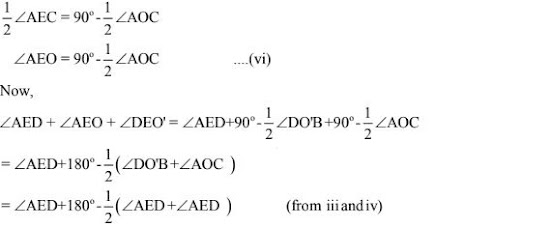= ∠AED + 180° – ∠AED
= 180°
So,
∠AED + ∠AEO + ∠DEO = 180°
So,
OEO' is straight line.
Hence, O, E and O' are collinear.

11. In Fig. 9.20. O is the centre of a circle of radius 5 cm, T is a point such that OT = 13 cm and OT intersects the circle at E. If AB is the tangent to the circle at E, find the length of AB.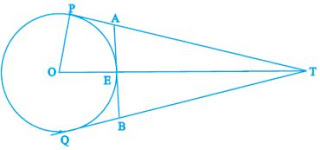Solution
OP is perpendicular to PT.
In ∆OPT,
OT2 = OP2 + PT2
⇒ PT2 = OT2 – OP2
⇒ PT2 = (13)2 – (5)2
= 169 – 25
= 144
⇒ PT= 12 cm
Since, the length of pair of tangents from an external point T is equal.
So,
QT= 12 cm
Now,
TA = PT – PA
⇒ TA = 12 -PA  ...(i)
and
TB = QT – QB
⇒ TB = 12 – QB  ...(ii)
Also,
PA = AE and QB = EB ...(iii) [Pair of tangents]
ET = OT – OE  [as, OE = 5 cm = radius]
⇒ ET = 13 – 5
⇒ ET = 8 cm
Since, AB is a tangent and OE is the radius.
OE ⊥ AB,
∠OEA = 90°
∠AET = 180° – ∠OEA  [Linear pair]
⇒ ∠AET = 90°
Now, in right angled ∆AET,
(AT)2 = (AE)2  + (ET)2  [by Pythagoras theorem]
⇒ (12 – PA)2  = (PA)2  + (8)2
On solving,
144 + (PA)2 – 24 PA = (PA)2  + 64
⇒ 24 PA = 80
⇒ PA = 10/3
So,
AE = 10/3
We join OQ,
Similarly,
BE = 10/3
Also,
AB = AE + BE
= 10/3 + 10/3
= 20/3

12. The tangent at a point C of a circle and a diameter AB when extended intersect at P. If ∠PCA = 110°, find CBA.
[Hint: Join C with centre O.]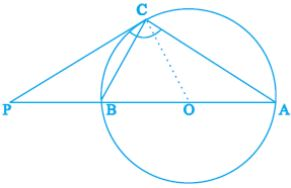Solution
Join OC. In this, OC is the radius .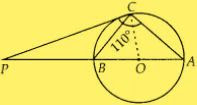We know that, tangent at any point of a circle is – perpendicular to the radius through the
point of contact.
Therefore,
OC ⊥ PC
Now,
∠PCA = 110° [Given]
∠PCO + ∠OCA = 110°
⇒ 90° + ∠OCA = 110°
⇒ ∠OCA = 20°
Also,
OC = OA = radius of circle
∠OCA = ∠OAC = 20°  [Sides opposite to equal angles are equal]
Since, PC is a tangent,
∠BCP = ∠CAB = 20°  [Angles in alternate segment]
In ∆PAC,
∠P + ∠C + ∠A = 180°
So,
∠P = 180° – (∠C + ∠A)
= 180°-(110°+ 20°)
= 180° – 130° = 50°
In ∆PBC,
∠BPC + ∠PCB + ∠CBP = 180°
⇒ 50° + 20° + ∠PBC = 180°
⇒ ∠PBC = 180° – 70°
⇒ ∠PBC = 110°
Since, ABP is a straight line.
Therefore,
∠PBC + ∠CBA = 180°
⇒ ∠CBA = 180° – 110° = 70°

13. If an isosceles triangle ABC, in which AB = AC = 6 cm, is inscribed in a circle of radius 9 cm, find the area of the triangle.
Solution
Join OB, OC and OA.
In ∆ABO and ∆ACO,
AB = AC  [Given]
BO = CO  [Radii of same circle]
AO = AO   [Common side]
∆ABO ≅ ∆ACO   [By SSS congruence criterion]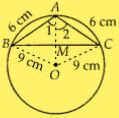∠1 = ∠2   [CPCT]
Now,
In ∆ABM and ∆ACM,
AB = AC  [Given]
∠1 = ∠2   [proved above]
AM = AM  [Common side]
So,
∆AMB ≅ ∆AMC  [By SAS congruence criterion]
∠AMB = ∠AMC  [CPCT]
Also,
∠AMB + ∠AMC = 180°  [Linear pair]
⇒ ∠AMB = 90°
We know that a perpendicular from the centre of circle bisects the chord.
So, OA is a perpendicular bisector of BC.
Let AM = x, then OM = 9 - x  [as, OA = radius = 9cm]
In right angled ∆AMC,
AC2 = AM2 + MC2  [By Pythagoras theorem]
⇒ MC2 = 6 – x2  ...(i)
In right angle ∆OMC,
OC2 = OM2 + MC2  [By Pythagoras theorem]
⇒ MC2 = 92 – (9 – x)2
From equation (i) and (ii),
62 – x2 = 92 – (9 – x)2
⇒ 36 – x2 = 81 – (81 + x2 – 18x)
⇒ 36 = 18x
x = 2
So,
AM = 2 cm
From equation (ii),
MC2 = 92 – (9 – 2)2
⇒ MC2 = 81 – 49 = 32
⇒ MC = 4√2 cm
So,
BC = 2MC
= 8√2 cm
Area of ABC = (1/2) × base  × height
= (1/2) × BC × AM
= (1/2) × 8√2 × 2
= 8√2  cm2
The required area of ΔABC is 8√2 cm2 .

14. A is a point at a distance 13 cm from the centre O of a circle of radius 5 cm. AP and AQ are the tangents to the circle at P and Q. If a tangent BC is drawn at a point R lying on the minor arc PQ to intersect AP at B and AQ at C, find the perimeter of the ∆ABC .
Solution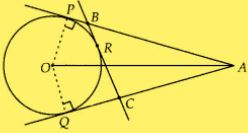We have,
∠OPA = 90°
[Tangent at any point of a circle is perpendicular to the radius through the point of contact]
In ∆OAP,
OA2 = OP2 + PA2
⇒ 132 = 52 + PA2
⇒ PA = 12 cm
Now,
Perimeter of ∆ABC = AB + BC + CA
= AB + BR + RC + CA
= (AB + BR) + (RC + CA)
= (AB + BP) + (CQ + CA)
[As, BR = BP, RC = CQ i.e., tangents from external point to a circle are equal]
Perimeter of ∆ABC = AP + AQ
= 2AP  [as, AP = AQ]
= 2 × 12
= 24 cm
Hence, the perimeter of ∆ABC = 24 cm.